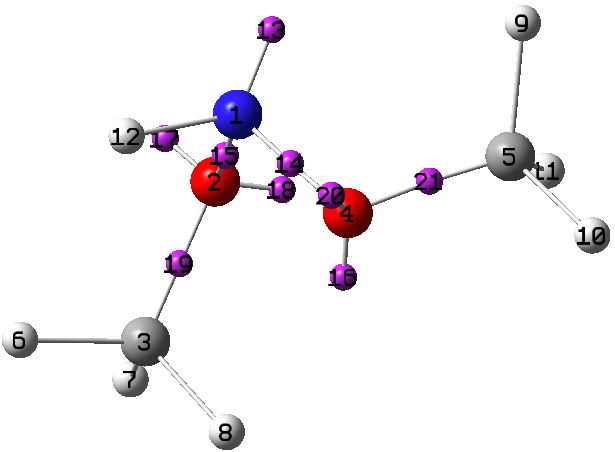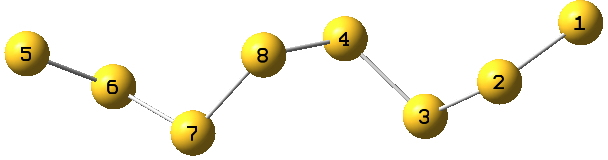## A periodic table for anomeric centres.

In the last few posts, I have explored the anomeric effect as it occurs at an atom centre X. Here I try to summarise the atoms for which the effect is manifest in crystal structures.

The effect is defined by X bearing two substituents, one of which Z is a centre bearing a “lone pair” of electrons (or two electrons in a π-bond), and another Y in which the X-Y bond has a low-lying acceptor or σ* empty orbital into which the lone pair can be donated. This donation will only occur if the Z-lone pair and the X-Y bond vectors align antiperiplanar. Here is the summary so far.

X Blog entry
B 16601
C 14508,8898
N this one
O 16646
Si 16601
P 16601
S this one

As required of a good periodic table, it has gaps that need completing, in this case X=N and X=S. Firstly N, for which the small molecule below is known (FUHFAP).A ωB97XD/Def2-TZVPP calculation yields an electron density distribution, which can be collected into monosynaptic basins using the ELF technique. There are two oxygen lone pairs (17 and 20) that are close to antiperiplanar to the adjacent N-O bond, having E(2) interaction energies obtained using the NBO method of 15.1 and 15.8 kcal/mol, typical of the anomeric range. The basin labelled 13 on X=N1 below is also perfectly aligned antiperiplanar with the adjacent O3-C2 bond, but its E(2) interaction energy is only 7.3 kcal/mol. Thus a strong anomeric interaction on the anomeric atom itself does not seem to occur. The same effect was noted for X=O in the previous post; the explanation remains unidentified.With the X=S gap, we have lots of opportunity with polysulfide compounds, a good example of which is the C2-symmetric and helical S8 dianion TEGWAFEach of the 8 sulfur atoms exhibits antiperiplanar orientation of an S lone pair with an adjacent S-S acceptor σ* orbital;
1:2-3=23.7 kcal/mol;
2:3-4=18.5;
3:4-8=11.7, 3:2-1=7.4;
4:8-7=11.4, 4:3-2=9.2.

This just surveys the central main group elements, and it is possible that this little mini-periodic table may yet grow.

## References

1. Henry S Rzepa., "C 2 H 7 N 1 O 2", 2016. http://dx.doi.org/10.14469/ch/195294
2. Rybak, W.K.., Cymbaluk, A.., Skonieczny, J.., and Siczek, M.., "CCDC 880780: Experimental Crystal Structure Determination", 2012. http://dx.doi.org/10.5517/CCYKJ88